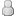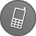• 11 Votes - 4.64 Average
• 1
• 2
• 3
• 4
• 5
01-11-2023, 01:50 PMPost: #391
 AxymeusJunior MemberPosts: 2 Joined: Dec 2022 Reputation: 0
So apparently the glitch I mentionned above is due to one cinematic rendering with an extra pixel in height, which breaks some shaders.

https://github.com/hrydgard/ppsspp/pull/12894

I have no doubt the team will update the built-in shaders to handle that properly, but I'd like to use a custom shader and I don't know how to update it... This is the current version, a port of a shader found in RetroArch.

Code:
```attribute vec4 a_position; attribute vec2 a_texcoord0; varying vec2 v_texcoord0; // vTexCoord varying vec2 v_texcoord1; // TextureSize uniform vec2 u_texelDelta; uniform vec4 u_setting; void main() {    v_texcoord0 = a_texcoord0;    v_texcoord1 = 1.0 / u_texelDelta;    gl_Position = a_position; }```

Code:
```#ifdef GL_ES precision mediump float; precision mediump int; #endif const float grid_strength = 0.04; const float target_gamma = 2.21; const float display_gamma = 2.2; const float saturation = 1.0; const float luminosity = 1.4; const float contrast = 1.0; const float rc = 0.98; const float gc = 0.795; const float bc = 0.98; const float rcg = 0.04; const float rcb = 0.01; const float gcr = 0.20; const float gcb = 0.01; const float bcr = -0.18; const float bcg = 0.165; uniform sampler2D sampler0; varying vec2 v_texcoord0;  // vTexCoord varying vec2 v_texcoord1;  // TextureSize float intsmear_func(float z) {    float z2 = z * z;    float z4 = z2 * z2;    float z8 = z4 * z4;    return z - 2.0 / 3.0 * z * z2 - 1.0 / 5.0 * z * z4          + 4.0 / 7.0 * z * z2 * z4 - 1.0 / 9.0 * z * z8          - 2.0 / 11.0 * z * z2 * z8 + 1.0 / 13.0 * z * z4 * z8; } float intsmear(float x, float dx) {    const float d = 1.5;    float zl = clamp((x - dx) / d, -1.0, 1.0);    float zh = clamp((x + dx) / d, -1.0, 1.0);    return d * (intsmear_func(zh) - intsmear_func(zl)) / (2.0 * dx); } void main() {      // LCD subpixel emulation    vec4 source_size = vec4(v_texcoord1, 1.0 / v_texcoord1);       vec2 texel_size = source_size.zw;    vec2 subtx_size = texel_size / vec2(3.0, 1.0);    vec2 range = source_size.xy / (vec2(1920, 1088) * source_size.xy);       float ltx = v_texcoord0.x - texel_size.x * 0.5;    float ttx = v_texcoord0.y + range.y;    float rtx = v_texcoord0.x + texel_size.x * 0.5;    float btx = v_texcoord0.y - range.y;       float subpix = mod(v_texcoord0.x / subtx_size.x + 1.5, 3.0);    float rsubpx = range.x / subtx_size.x;    vec4 lcol = vec4(          intsmear(subpix + 1.0, rsubpx),          intsmear(subpix, rsubpx),          intsmear(subpix - 1.0, rsubpx),          0.0);    vec4 rcol = vec4(          intsmear(subpix - 2.0, rsubpx),          intsmear(subpix - 3.0, rsubpx),          intsmear(subpix - 4.0, rsubpx),          0.0);             vec2 tl0 = (floor(vec2(ltx, ttx) / texel_size) + 0.5) * texel_size;    vec2 tr0 = (floor(vec2(rtx, ttx) / texel_size) + 0.5) * texel_size;    vec2 bl0 = (floor(vec2(ltx, btx) / texel_size) + 0.5) * texel_size;    vec2 br0 = (floor(vec2(rtx, btx) / texel_size) + 0.5) * texel_size;    vec4 tlc = pow(texture2D(sampler0, tl0), vec4(display_gamma)) * lcol;    vec4 trc = pow(texture2D(sampler0, tr0), vec4(display_gamma)) * rcol;    vec4 blc = pow(texture2D(sampler0, bl0), vec4(display_gamma)) * lcol;    vec4 brc = pow(texture2D(sampler0, br0), vec4(display_gamma)) * rcol;       vec2 border = floor((v_texcoord0.st / subtx_size) + 0.5);    vec2 bordet = clamp((border + vec2(0.0, +grid_strength)) * subtx_size,          vec2(ltx, btx), vec2(rtx, ttx));    vec2 bordeb = clamp((border + vec2(0.0, -grid_strength)) * subtx_size,          vec2(ltx, btx), vec2(rtx, ttx));       vec4 average_color;    float total_area = 2.0 * range.y;    average_color  = ((ttx - bordet.y) / total_area) * tlc;    average_color += ((bordeb.y - btx) / total_area) * brc;    average_color += ((bordeb.y - btx) / total_area) * blc;    average_color += ((ttx - bordet.y) / total_area) * trc;       vec4 current = pow(average_color, vec4(1.0 / display_gamma));       // Color regrading to simulate the LCD display    vec4 screen = pow(current, vec4(target_gamma));    vec4 avglum = vec4(0.5);    screen = mix(screen, avglum, (1.0 - contrast));       mat4 color = mat4(          rc, rcg, rcb, 0.0,          gcr, gc, gcb, 0.0,          bcr, bcg, bc, 0.0,          0.0, 0.0, 0.0, 0.0);             float rad = (1.0 - saturation) * 0.3086;    float gad = (1.0 - saturation) * 0.6094;    float bad = (1.0 - saturation) * 0.0820;    mat4 adjust = mat4(          rad + saturation, rad, rad, 1.0,          gad, gad + saturation, gad, 1.0,          bad, bad, bad + saturation, 1.0,          0.0, 0.0, 0.0, 1.0);             color = color * adjust;    screen = clamp(screen * luminosity, 0.0, 1.0);    screen = color * screen;       gl_FragColor = pow(screen, vec4(1.0 / display_gamma)); }```

Maybe someone can help me with that? The shader also assumes an output resolution of 1920x1088 (4x the PSP resolution) but it's hard-coded. It would be better to use the display size.

I'm not big on programming and just managed to make this one work lol. Any help is appreciated thank you.
02-18-2023, 03:11 PMPost: #392
 el_rikaJunior MemberPosts: 28 Joined: Mar 2016 Reputation: 0
Thank you Luna for all you efforts !

If you have the time man, could you add the fxaa code to the zoom/bloom shader combo, if possible?

https://we.tl/t-16sbVNpIB6

(they are speciffically for Re4 in Dolphin, but you are the one that helped me before (devs on Dolphin are not very active in forum), so i thought i'd give it a shot again).

Thank you friend!
02-18-2023, 04:32 PMPost: #393
 LunaMooPosting FreakPosts: 1,789 Joined: Sep 2013 Reputation: 159
You don't need to mix effects together for PPSSPP anymore, PPSSPP supports multi pass shaders and you can freely mix effects as you wish and do it directly from UI.
PPSSPP UI even includes shader parameters/settings as long as the author of the shader included some. Post process effects were moved not long ago to Display Layout & Effects screen where you can freely select and mix many effects together.

http://forums.ppsspp.org/showthread.php?tid=3590&pid=117172#pid117172 - simple CE scripts to help creating CWCheats,
https://github.com/LunaMoo/PPSSPP_workarounds - CWCheat workarounds.
02-18-2023, 09:00 PMPost: #394
 el_rikaJunior MemberPosts: 28 Joined: Mar 2016 Reputation: 0
Oh, i know that in ppsspp the shader system works like that, and it's amazing.

However, i am trying to use that shader combo in Dolphin, which doesn't support multi layered shaders.
The idea was to have all 3 (zoom, bloom, fxaa) in a single file.
Zoom and Bloom are already there (also made by you, a few years back, and i thank you again for it), what's missing is Fxaa.

Please ignore my request if i'm being out of line good sir.
03-25-2023, 01:39 AMPost: #395
 kingofcheezwizJunior MemberPosts: 1 Joined: Mar 2023 Reputation: 0
I'm trying to add these shaders to the linux flatpak on Steam Deck. I found the default shaders directory. It is located at /var/lib/flatpak/app/org.ppsspp.PPSSPP/current/active/files/share/ppsspp/assets/shaders/ but I have not found a way to enable write access to this folder. Is there any way to add these to the this version of PPSSPP?
03-25-2023, 09:08 PMPost: #396
 LunaMooPosting FreakPosts: 1,789 Joined: Sep 2013 Reputation: 159